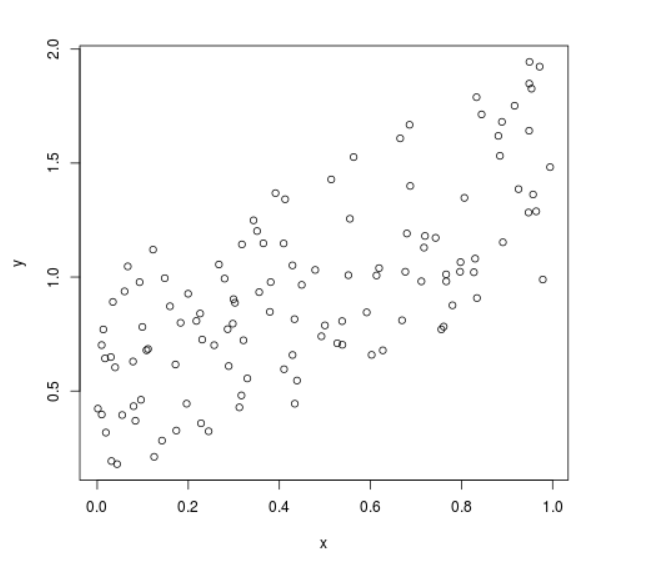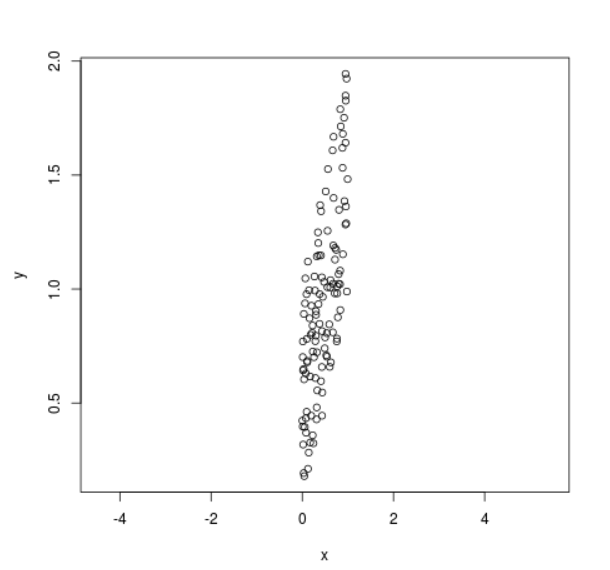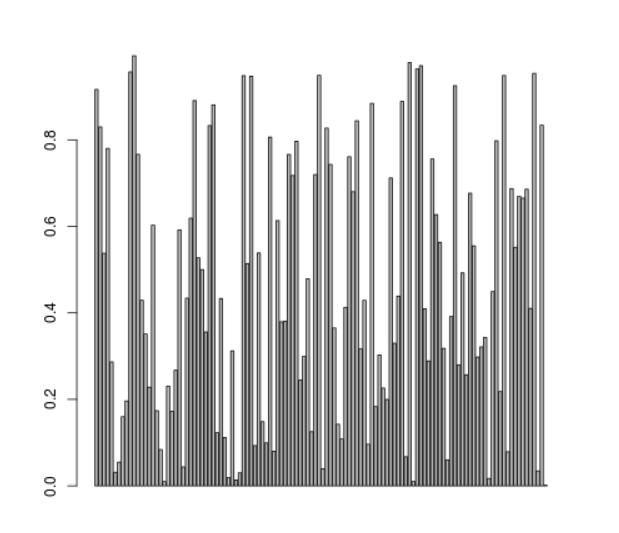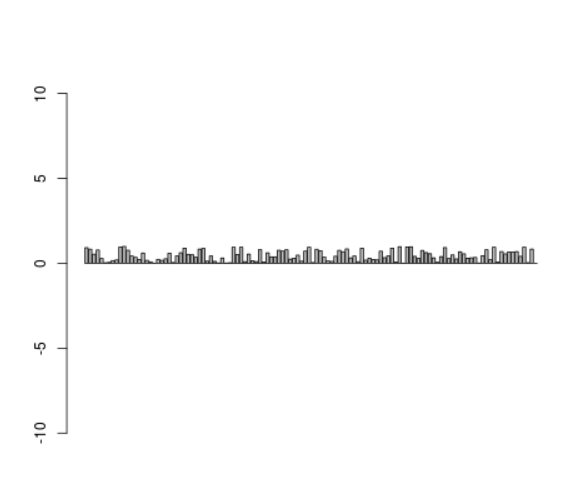Open in App
Not now

# Set Aspect Ratio of Scatter Plot and Bar Plot in R Programming – Using asp in plot() Function

• Last Updated : 30 Jun, 2020

`asp` is a parameter of the `plot()` function in R Language is used to set aspect ratio of plots (Scatterplot and Barplot). Aspect ratio is defined as proportional relationship between width and height of the plot axes.

Syntax: plot(x, y, asp )

Parameters:
x, y: Coordinates of x and y axis
asp: Aspect ratio

Example 1:

 `# Set seed for reproducibility``set.seed``(86000)     `` ` `# Create random x variable``x <- ``runif``(120)     `` ` `# Create y variable correlated with x``y <- x + ``runif``(120)      `` ` `# Plot without setting aspect ratio``plot``(x, y)       `` ` `# Plot with asp = 5``plot``(x, y, asp = 5)         `

Output:

• Plot Without Aspect Ratio:• Plot with Aspect Ratio:Example 2:

 `# Set seed for reproducibility``set.seed``(86000)              `` ` `# Create random x variable``x <- ``runif``(120)              `` ` `# Create y variable correlated with x``y <- x + ``runif``(120) `` ` `# Regular barplot``barplot``(x)                   `` ` `# Barplot with aspect ratio of 5``barplot``(x, asp = 5)         `

Output:

• Bar Plot Without Aspect Ratio:• Bar Plot with Aspect Ratio:Here, the asp option increases the width of the y-axis.

My Personal Notes arrow_drop_up